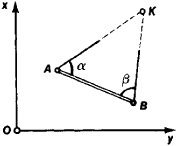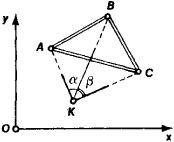# Intersection, Geodetic

## Intersection, Geodetic

a method for determining the position of a point (a reference point in geodesy or a gun or target in artillery) by measuring the lengths of the segments connecting the point with certain given points or by measuring the angles between the directions of the segments. De-pending on the type of quantity measured, a distinction is made between linear and angular geodetic intersections.

To determine the position of a point K in space using a linear geodetic intersection, it is necessary to measure at least the lengths Si of the three segments that connect this point with the three points A, B, and C with given coordinates (Figure 1). Then the coordinates of the unknownFigure 1. Three-dimensional geodetic intersection

point may be found from the solution of a system of equations of the type

Si2 = (Xi − X)2 + (Yi − Y)2 + (Zi − Z)2

where i = 1, 2, 3 or A, B, C. If the unknown point lies on the surface of a reference ellipsoid or on a plane, the measurement of the lengths of the two segments that connect it to two known points is sufficient to determine its position by a linear geodetic intersection.

The determination of the position of a point in space by using an angular geodetic intersection involves the determination of the direction cosines of the two lines mentioned above; this is accomplished by measuring the zenith distances and azimuths of the point at the corresponding known points.

The angular intersection of a point at the surface of the reference ellipsoid or on the plane is subdivided into the intersection and the resection. To determine the position of a point K by geodetic intersection, it is sufficient to measure the two angles a and β of the triangle ABK at the known points A and B (Figure 2), but in a geodetic resection it is necessary to measure at the point the two angles between theFigure 2. Angular geodetic intersectionFigure 3. Angular geodetic intersection

directions to the three assigned points A, B, and C (Figure 3). The position of the point is determined from the trigonometric relationships that link the measured angles and distances between the known points.

Various combinations of geodetic intersection and resection are also used in practical geodetic work. Here a number of quantities that is larger than necessary is measured. The position of the unknown point is determined from the corresponding equating calculations.

### REFERENCES

Chebotarev, A. S. Geodeziia, 2nd ed., parts 1–2. Moscow, 1955–62.
Veis, G. Geodezicheskoe ispol’zovanie iskusstvennykh sputnikov Zemli. Moscow, 1967. (Translated from English.)
Site: Follow: Share:
Open / Close